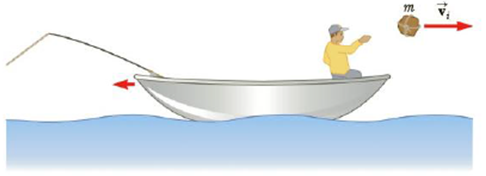Chapter 6, Problem 26P

Chapter
Section
Textbook Problem

A 75-kg fisherman in a 125-kg boat throws a package of mass m = 15 kg horizontally toward the right with a speed of vi = 4.5 m/s as in Figure P6.26. Neglecting water resistance, and assuming the boat is at rest before the package is thrown, find the velocity of the boat after the package is thrown.Figure P6.26

To determine
Velocity of the boat.

Explanation

Given Info: Mass of the fisherman is mF=75kg , Mass of the boat is mB=125kg , Mass of the package is mP=15kg and mass of boat and fisherman is mBF=200kg .

Velocity of the package thrown is positive towards the right is vP=+4.5m/s

Initial velocities of the package thrown, boat and fisherman are vP,vB,vF=0

Apply conservation of momentum to boat fisherman –package system is

(mBFvBF+mPvP)i=(mBFvBF+mPvP)f (1)

• mP is the  mass of the package
• mBF is the mass of the Boat and fisherman
• vP is the velocity of the package thrown
• vBF is the velocity of the package thrown

Use initial velocities in equation (1) and solve for vBF

Still sussing out bartleby?

Check out a sample textbook solution.

See a sample solution

The Solution to Your Study Problems

Bartleby provides explanations to thousands of textbook problems written by our experts, many with advanced degrees!

Get Started